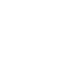# ACT & SAT Question of the Day: May 7, 2014

If you are reading this in an email you received from me, do not click the link to sat.collegeboard.org below. Use the link to my website that is farther down on the email.

http://sat.collegeboard.org/practice/sat-question-of-the-day?questionId=20140507&oq=1 (This link takes you to today’s question. If you use my archive, you will see the question related to my SAT explanation for that date.)

Reading this blog is 10% about learning how to answer today’s questions and 90% learning how to apply strategies and analyze questions you may see on test day.

The answer is E.  This is one of those questions when the math is easy but you have to understand the words in the word problem!  By the “nth term,” they simply mean the “number of the term.”  For example, when n is 1, they mean the 1st term.  Then when n is 2, they mean the second term and so on.

The question is pretty easy when you just pick a value for n and plug it into the answers.  Let’s use the 3rd term.  So n = 3.  They tell us the 3rd value in the sequence is 11; so, when n = 3 the equation has to result in 11.  Plugging in 3 for n for each of the answers, only Answer E works.  All done.

This question reminds me of something you should do as you prepare for the math test.  Take the free math diagnostic on my website.  Here’s the link: https://maxthetest.com/free-help/math-diagnostic;oq=1.  Be sure to save the web page because you may want to do only a part of the diagnostic at a time.  For example, you could do the arithmetic and algebra sections and then later on do geometry and miscellaneous math.

Students tell me it takes about 45 minutes.  If you are only taking the SAT, just do the SAT diagnostic.  If you are taking the ACT or both tests, do the ACT diagnostic.  When you are finished, you will get a list of the math concepts that show up on the SAT and/or ACT.  You will also see which math concepts you need to review.

What you will notice as you practice is that you got a lot of the questions right but you miss actual SAT or ACT questions that require that knowledge!  That’s because the difficulty of the question doesn’t lie in the math itself but rather in the way they ask questions.  That explains why you hear me tell you that practice is critical.  You have to become familiar with the way they ask questions.  For example, the “nth term.”  Now you know what that means.

Keep working and send me an email with any questions you have.

Let’s see what the ACT folks have for us today.

The answer is B.  As for every reading test question you’ll ever see, simply remember to use PICK.  The “I” stands for Insertable.  Ask yourself, “Does this answer insert or fit into the passage without changing anything?”  If an answer adds to or disagrees with the passage when you insert it, it is a wrong answer.  When you do so with the answers for today’s question, you can easily eliminate the three wrong answers.  They all disagree or add.

QotD Words of “Wiz-dom”:

Keep in mind the best strategy for the reading test is to focus on eliminating wrong answers.  Many students make the mistake of trying to justify keeping an answer.  That’s a very bad approach.  You end up wasting a lot of time and talking yourself into wrong answers.

It is much more efficient to justify why an answer is wrong.  For a full explanation of PICK and my other reading strategies, read my free website or watch Video #305.

Make your day a positive one.

Bob Alexander, the “SAT and ACT Wizard”